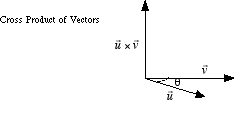index: click on a letter A B C D E F G H I J K L M N O P Q R S T U V W X Y Z A to Z index index: subject areas numbers & symbols sets, logic, proofs geometry algebra trigonometry advanced algebra & pre-calculus calculus advanced topics probability & statistics real world applications multimedia entrieswww.mathwords.com about mathwords website feedback

 Cross Product A way of multiplying two vectors, written u × v, in which the product is another vector. The cross product of two vectors results in a vector which is orthogonal to both the vectors being multiplied. The magnitude of the cross product of two vectors is found by the formula |u × v| = |u| |v| sin θ, where θ is the smaller angle between the vectors. Note: Cross products are not commutative. That is, u × v ≠ v × u. The vectors u × v and v × u have the same magnitude but point in opposite directions.See also